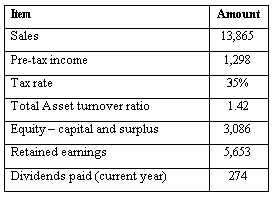### CFA Practice Question

There are 534 practice questions for this study session.

### CFA Practice Question

An analyst has extracted the following information from the financial statements of Myco:What is the leverage ratio (total assets/equity) for Myco?
A. 2.253
B. 1.117
C. Can't say
Explanation: There are two ways to calculate this:

Method 1: TA / Eq = (TA / Sales) x (Sales / Eq) = (1/1.42) x (13,865 / 8,739) = 1.117

Method 2: DuPont formula - ROE = (Net income / Sales) x (Sales / Assets) x (Assets / Equity)

By rearranging, Assets / Equity = 0.0965 / (0.0609 x 1.42) = 1.116 (rounding error)

User Comment
Shelton Method 2 is unuseful under time-pressure:
ROE = NI/Eq = (NI/Sale)*(Sale/Asset)*(Assets/Eq) <=>
Leverage = Assets/Eq = (Ass/Sale)*(Sale/Eq) = Ass.To^(-1) * [Sale /(RE+Cap.Surplus)]
gaur I disagree with method 1. Asset Turnover ratio is Net Sales / AVERAGE Assets. By putting this into the equation we get 13865/x = 1.42 therefore, x = 9764.08 which is AVERAGE Assets and not Total Asset which should be the EO year balance of Assets.
adeelj gaur Method 1 still works
1.42= 13865/x , then x= 9764.08 divide this number by total equity which is (RE+Cap.Surplus)= 8739. That is 9764.08/8739 = 1.117
dimanyc What is Equity-Capital and Surplus? and why do we not subtract Dividends from (Eq+Ret'd Earn)?
ManuB Why is 8,739 the equity? Why do we have to add the retained earnings to "Equity - capital and surplus"?
Tatyana Dividends are already substracted from net income to get retained earnings.
Equity = capital and surplus + retained earnings = 3086 + 5653 = 8739
staudinger much easier is ..
Equity = 3086 + 5653
total assets = 13865 / 1.43

and then TA / Equity ...
serboc GREAT QUESTION!!
ofabian i did it the way staudinger did it.
najat me too, much easier especially given the time we have!
bidisha The way I memorized DuPont:
Assets/equity: How much assets were you able to generate from the capital that owners put in
Sales/assets: how much sales/revenue did you generate from the assets you had
NI/sales: how much of the revenue was left over as net income for the owners.
CJPerugini The financial leverage ratio is taken at a snippet in time, not over a period of time. Asset turnover is not of use because it is based on average total assets. There is no way to determine end of period assets from the given information.